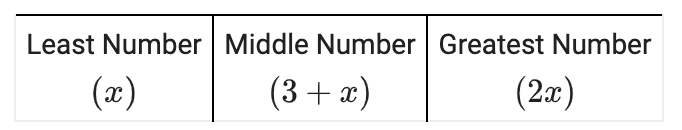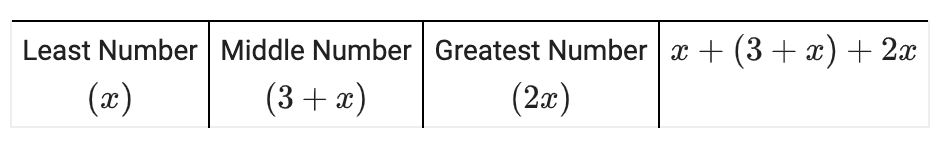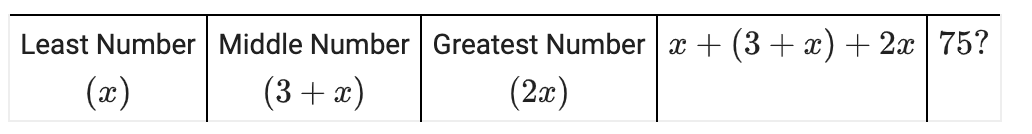### Home > AC > Chapter 1 > Lesson 1.2.2 > Problem1-55

1-55.

Solve the problem below using Guess and Check. State your solution in a sentence.

Jabari is thinking of three numbers. The greatest number is twice as large as the least number. The middle number is three more than the least number. The sum of the three numbers is $75$. Find the numbers.

Rewrite the word problem as an equation or a system of equations.

Let the least number equal $x$.

A row with 3 columns labeled, left to right, as follows: Least number, x. Middle number, 3 + x. Greatest number, 2 x.

Fourth column added, labeled, x + (3 + x) + 2 x.Make a guess for what $x$ could be. Was your answer higher or lower than $75$? Adjust your next guess accordingly.